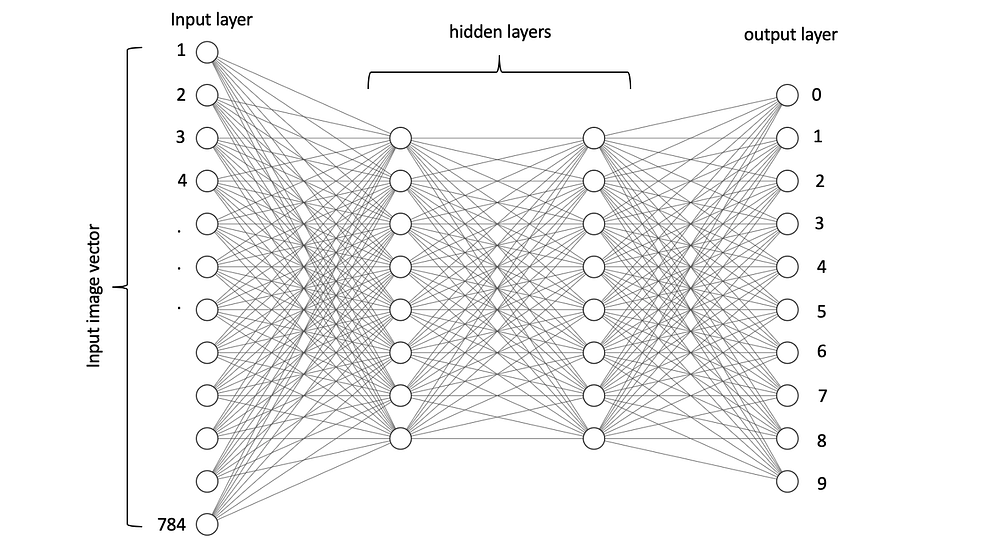# Under the Hood of Deep Learning

Source: Deep Learning on MediumWhere did all these numbers come from? Well, the input nodes were already given, Z is the result for multiplying the input activations with the weights and add them together, y^ is the result for multiplying Z with its weight. Finally, y is also given which is the desired output. Generally, to compute any output, we multiply the activations with the weights and add them together. This the formula for the first layer.